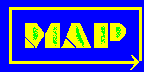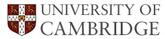# Materials Algorithms ProjectUtilities: Subroutines LibraryA library of SUBROUTINES providing a range of general purpose utilities.

Format of documentation within this library.

[A][B][C][D] [E][F][G][H] [IJ][K][L][M] [N][O][PQ][R] [S][T][UVW][XYZ]

### A

MAP_UTIL_ANALY
A linear regression subroutine which determines the intercept and gradient of the best fit line between two variables, together with the correlation coefficient.
Language: FORTRAN

[Top]

### B

MAP_UTIL_BOUND
This subroutine writes out an error message when an input variable is out of bounds and then requests a new value.
Language: FORTRAN

[Top]

### C

MAP_UTIL_CROSS
Takes a vector cross product and converts all vectors to real space.
Language: FORTRAN

[Top]

### D

MAP_UTIL_DET
Calculates the determinant of a 3x3 matrix.
Language: FORTRAN

[Top]

[Top]

### F

MAP_UTIL_FDHEAT
Model of the  induction heating and water spray quenching of a plate or cylinder of steel. The heat flow equation is integrated numerically using the Crank-Nicholson method.
Language: FORTRAN

[Top]

[Top]

[Top]

### IJ

MAP_UTIL_INDHEAT
Simplified model of the  induction heating and water spray quenching of a plate of steel. The heat flow equation had been integrated analytically and this program evaluates the solved equations for different heat inputs.
Language: FORTRAN

MAP_UTIL_INVERS
Calculates the inverse of a 3x3 matrix (held in a vector form).
Language: FORTRAN

[Top]

[Top]

[Top]

### M

MAP_UTIL_MAGG
Calculates the inverse of the magnitude of the product of two vectors. The second vector is formed by a matrix transformation on the first vector.
Language: FORTRAN

[Top]

### N

MAP_UTIL_NORM
Normalises a three dimensional vector.
Language: FORTRAN

[Top]

### O

MAP_UTIL_ODD
Tests if a number is odd or even.
Language: FORTRAN

[Top]

### PQ

MAP_UTIL_POLY
Calculates a least squares fit of a second order polynomial to an (x,y) data set.
Language: FORTRAN

MAP_UTIL_PROD
Calculates the product of two 3x3 matrices.
Language: FORTRAN

[Top]

### R

MAP_UTIL_REED
Traps typing errors in real data input.
Language: FORTRAN

MAP_UTIL_REEDI
Traps typing errors in integer data input.
Language: FORTRAN

MAP_UTIL_ROT
Calculates a three dimensional rotation matrix.
Language: FORTRAN

[Top]

### S

MAP_UTIL_SORT3
Sorts the elements of a two dimensional array.
Language: FORTRAN

[Top]

### T

MAP_UTIL_TRANS
Multiplies a 3x3 matrix by a 3-d column vector.
Language: FORTRAN

MAP_UTIL_TRANS2
Multiplies a 3-d row vector by a 3x3 matrix.
Language: FORTRAN

MAP_UTIL_TRAPE
Integrates a function which is specified numerically using a trapezoidal rule.
Language: FORTRAN

[Top]

[Top]

[Top]